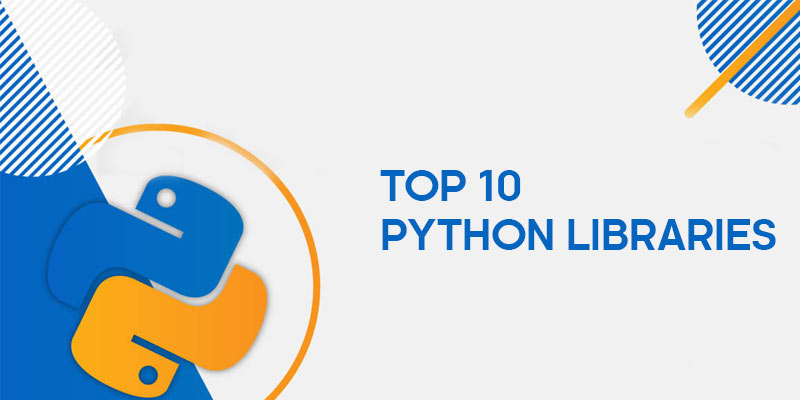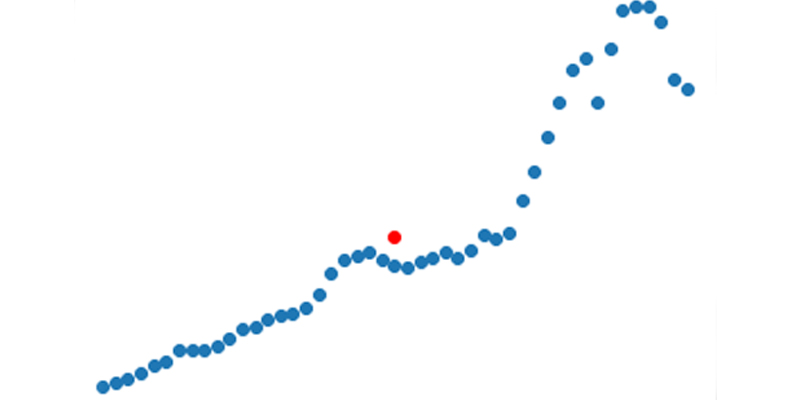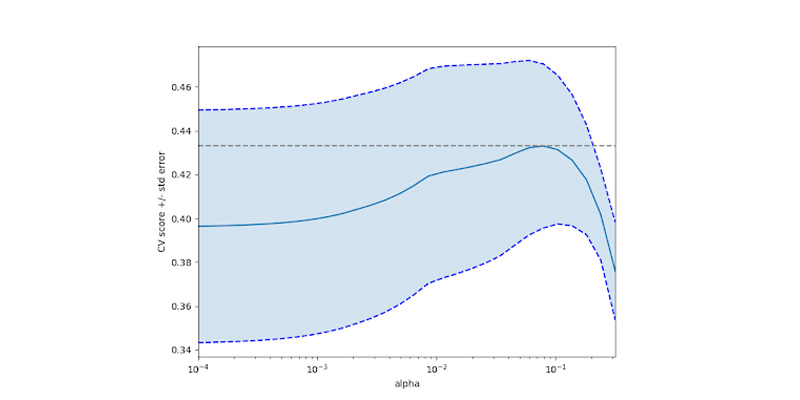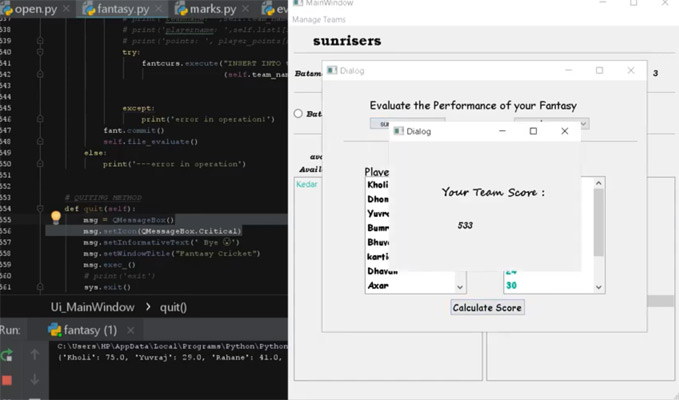In this blog, I will talk about the famous 10 libraries of python with their features along with examples.

Opencv Python
BeautifulSoup
Requests
Numpy
Scikit Learn
Tensorflow
Matplotlib
SqlAlchemy
PyQt

### Opencv PythonOpencv or Open Source Computer Vision is a library for image processing, machine learning, and computer vision applications, etc. originally developed by Intel.

What You Can Do With OpenCV

Process images and videos to identify objects
Document field detection
Detection of specific color
Detect edges of an image
Cartoonize an image
Facial Landmarks and Face detection
Camera calibration and 3D reconstruction

Image blurring with Opencv Python

Here is an example of blurring an image using gaussian blurring with OpenCV library.

 import cv2 import numpy as np   image = cv2.imread(‘got.png’)   cv2.imshow(‘Real Image’, imag) cv2.waitKey(0)   # Gaussian Blurring gauss = cv2.GaussianBlur(image, (8, 8), 0) cv2.imshow(‘gaussian blurring’, Gaussian) cv2.waitKey(0) cv2.destroyAllWindows()

Output for the above programWith this documentation, you can start making your own detection models.

### Tensorflow

Google Brain team’s, Tensorflow can be used to implement machine learning, deep learning, or neural network applications. Tensorflow is most famous because of its processing distribution between GPUs and CPUs. Tensorflow supports high level APIs and low level APIs for distribution.

A tensor can be described as an array of 0,1,2,3 or a higher dimensional array with homogenous data, upon which the scientific calculations are done just like numpy arrays with three properties as shape, size, and type.

With Tensorflow you can create

Convolution Neural Networks(CNNs)
Natural Language Processing(NLP)
Recurrent Neural Network(RNN)

Example of creating tensor in Tensorflow

 # Program to create tensor in tensorflow   import tensorflow as tf with tf.compat.v1.Session() as sess:      x = tf.range(12.0, 100.0, delta = 25.5)      y= tf.range(80.0, delta = 25.5, name =”y”)      print(x)      print(sess.run(x))      print()      print(y)      print(sess.run(y))

Output for the above program

 Tensor(“range_1:0”, shape=(4,), dtype=float32) [12. 37.5 63. 88.5] Tensor(“y_1:0”, shape=(4,), dtype=float32) [ 0. 25.5 51. 76.5]

### Matplotlib

Matplotlib is a 2-D plotting or data visualization library, originally written by John D. Hunter. Matplotlib can be used to embed graphs, diagrams, or plots in web applications with flask, Django, or desktop applications such as Pyqt, Tkinter,wxpython, etc.

What you can plot with matplotlib

bar charts
pie charts
Histograms
Scatter plots
error charts
Power spectra
Stem plots

And all other charts that you want to visualize..

Example of plotting scatter plot using Matplotlib

 import pandas as pd import matplotlib.pyplot as plt %inline matplotlib # to prevent opening of plot in a new window df = pd.read_csv(‘percapita.csv’) # reading data from a csv file plt.xlabel(‘years (1960-2016’)) # labeling x axis plt.ylabel(‘per capita (dollars)’) # labeling y axis plt.scatter(df.year,df.per_capita,color=blue) # plotting mean values of year and capital plt.scatter(np.mean(df.year),np.mean(df.per_capita),color=’red’)

Output for the above program

<matplotlib.collections.PathCollection at 0x7f3d8322e898>### Numpy

Numpy or Numerical python is a free and open-source library for working with n-dimensional arrays or ndarrays. It is often used with other libraries such as scipy, matplotlib, Pandas, scikit for scientific computations for various data science or machine learning applications. Numpy is partly written in Python and the rest with C and C++.

Use Of Numpy

Easily working with arrays of high dimension.
Mathematical operations can be effortlessly applied.
Applying statistical implementations across arrays.
Widely used in data science, machine learning projects.

Example for converting an array of temperatures in Celsius to Fahrenheit using numpy.

 cel_arr = np.array([20.2,20.4,22.9, 21.5,23.7, 25.3,21.8,24.2,20.9, 22.1]) print(cel_arr) feh_arr = cel_arr * (9 / 5) + 32 print(feh_arr)

Output for the above program

 [20.2 20.4 22.9 21.5 23.7 25.3 21.8 24.2 20.9 22.1] [68.36 68.72 73.22 70.7 74.66 77.54 71.24 75.56 69.62 71.78]

### Scikit Learn

Scikit Learn is a python library mostly used along with numpy and pandas for working with complicated or complex data.

It is used for cross validations such as checking the precision of unsupervised and supervised models with various algorithms.

Scikit learn is used for

Classification, grouping, or sorting of datasets.
Clustering and Model selection
For Regressions such as Linear, Logistic, Multiple, or binomial regression.
Extracting objects or features from images and documents.

Here is an example from scikit-learn exercises for cross-validation of the diabetes dataset.

 import numpy as np import matplotlib.pyplot as plt from sklearn import datasets from sklearn.linear_model import LassoCV from sklearn.linear_model import Lasso from sklearn.model_selection import KFold from sklearn.model_selection import GridSearchCV X, y = datasets.load_diabetes(return_X_y=True) X = X[:150] y = y[:150] lasso = Lasso(random_state=0, max_iter=10000) alphas = np.logspace(-4, -0.5, 30) tuned_parameters = [{‘alpha’: alphas}] n_folds = 5 clf = GridSearchCV(lasso, tuned_parameters, cv=n_folds, refit=False) clf.fit(X, y) scores = clf.cv_results_[‘mean_test_score’] scores_stdv = clf.cv_results_[‘std_test_score’] plt.figure().set_size_inches(8, 6) plt.semilogx(alphas, scores) std_error = scores_stdv / np.sqrt(n_folds) plt.semilogx(alphas, scores + std_error, ‘b–‘) plt.semilogx(alphas, scores – std_error, ‘b–‘) plt.fill_between(alphas, scores + std_error, scores – std_error, alpha=0.2) plt.ylabel(‘CV score +/- std error’) plt.xlabel(‘alpha’) plt.axhline(np.max(scores), linestyle=’–‘, color=’.5′) plt.xlim([alphas, alphas[-1]]) plt.show()

Output for the above programCross validation is a technique for testing the effectiveness of a model and to evaluate if we have enough data.

Check out this Complete Online Data Science Course by FITA, which includes Supervised, Unsupervised machine learning algorithms, Data Analysis Manipulation and visualization, reinforcement testing, hypothesis testing, and much more to make an industry required data scientist at an affordable price, which includes certification, support with career guidance assistance with an active placement cell, to make you an industry required certified data scientist.

### Requests

Requests is one of the famous libraries for making Http requests using python, for human beings.

Requests is used in

Read the response from the requested url.
Send a GET, POST, PUT, DELETE requests with its methods.
Handle exceptions.
Customize headers and data of the url.

Example program for scraping the FITA website with requests.

 import requests x = requests.get(‘https://www.fita.in/’) print(x.status_code) # output: 200 print(x.headers[‘Date’]) # output: Wed, 23 Sep 2020 16:02:02 GMT print(x.headers[‘Keep-alive’]) # output: timeout=5, max=100
 print(x.text)

Output for the above program

 FITA : Java, Hadoop, Android, AngularJS, Selenium, Software Testing, PHP, German, Salesforce, SEO, AngularJS, AWS, Cloud Computing, RPA, DevOps, IoT, Blockchain, Data Science, Digital Marketing, Python, Ethical Hacking, Dot Net Training in Chennai, Coimbatore, Madurai & Bangalore ….

Check out this Online Python Course by FITA. FITA provides a complete Python course that covers all the beginning and the advanced concepts of python including Django, along with hands on building real-time projects like Bitly and Twitter using Django Framework, along with placement support, and certification at an affordable price with an active placement cell, to make an industry required certified python and Django developer.

### Beautiful Soup

Beautiful soup is a python library for parsing data from the websites or for web scraping. It removes all those tags and styles from the source code and only uses the data that we want without having to reload the page, like the search for a <a> tag and return only its href value.

Scraping data with Beautiful Soup involves the following steps

Get the URL of the site.
Send a request to the server
Read the response from the server
Inspect the page and select elements you want
Parse using a scraper and store the data.

Example program for scraping all the courses available at FITA using Beautiful Soup

 import requests from bs4 import BeautifulSoup url = requests.get(‘https://www.fita.in’) page = url.content soup = BeautifulSoup(page,’html.parser’) links = soup.find_all(‘div’,class_=’course-name’) for i in links: link = i.find(‘h4’) print(link.text.strip())

Output

Well, you can surely grab any of these courses at an offline or online mode with this link at an affordable price.

### sqlalchemy

Sqlalchemy is a famous ORM or Object Relational mapper for Python, which converts the user defined classes to SQL database or tables. All the operations of raw SQL can be performed using Python classes(inheriting models from sqlalchemy) and will be mapped to the databases.

Companies using Sqlalchemy

FreshBooks
WeGetFinancing
DropBox
The OpenStack Project
Yelp!
Survey Monkey

Example of creating a table with sqlalchemy

 from sqlalchemy import create_engine from sqlalchemy.ext.declarative import declarative_base engine = create_engine(‘sqlite:///:memory:’, echo=True) Base = declarative_base() from sqlalchemy import Column, Integer, String class User(Base): __tablename__ = ‘Friends’ id=Column(Integer, primary_key=True) fullname = Column(String) nickname = Column(String) def __repr__(self): return “” % ( self.fullname, self.nickname)

Which will create a table as follows

 Table(Friends’, MetaData(bind=None), Column(‘id’, Integer(), table=, primary_key=True,nullable=False), Column(‘fullname’, String(), table=), Column(‘nickname’, String(), table=), schema=None)

### PyQt5

Pyqt5 is a library for creating desktop applications or interacting programs using GUI.PyQt5 is the latest version of pyQt, it lets you use the Qt GUI framework and Qt designer for making the layout of the application. An alternative to Pyqt would be Tkinter, which is lightweight and lets the developer decide the layout and components.

Here is an example picture of an application I made with pyqt for cricket score evaluation.You can have a glimpse of the source code for this application here

Pytest: Helps you write a better program

Whether you are writing a small program or a complex one, a program for deployment or for development, testing the program is important in every stage, therefore pytest can help you code failures, fixtures, and much more.

Features of Pytest

Automatically find and run tests.
Supports parallel testing
Simple but powerful fixture model
Can generate test reports in various forms (html report,json report, etc)

You can find the full documentation of pytest here.

To get in-depth knowledge of Python along with its various applications and real-time projects like twitter and bitly clone with Django, you can enroll in Python Training in Chennai or Python Training in Bangalore by FITA, which covers all the basics and advanced concepts of python including exception handlings, regular expressions, along with building real-time projects like Bitly and Twitter with Django, or enroll for a Data science course at Chennai or Data science course in Bangalore which includes Supervised, Unsupervised machine learning algorithms, Data Analysis Manipulation and visualization, reinforcement testing, hypothesis testing and much more to make an industry required data scientist at an affordable price, which includes certification, support with career guidance assistance and an active placement cell, to make you an industry required certified data scientist and python developer.

﻿